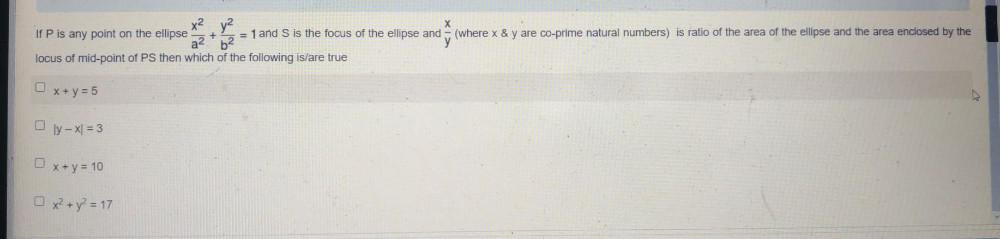Question:

# XI x2 y2 a 2 If P is any point on the ellipse + = 1 and S is the focus of the ellipse and b2 locus of mid-point of PS then whichXI x2 y2 a 2 If P is any point on the ellipse + = 1 and S is the focus of the ellipse and b2 locus of mid-point of PS then which of the following is/are true (where x & y are co-prime natural numbers) is ratio of the area of the ellipse and the area enclosed by the x + y = 5 0 ly - x = 3 x + y = 10 x2 + y2 = 17